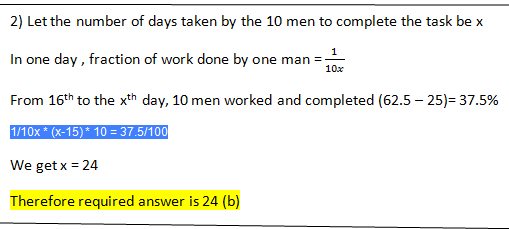New Students Offer - Use Code HELLO

# Maths Quiz For SBI PO Pre 2017: Part 6### Question 1

Solution A is a solution with 10% concentration while solution B is a solution with x% concentration and solution C is a solution with x²% concentration. If A and B are mixed in some ratio m:n, we get a solution with 6% concentration while if A and C are mixed in the same ratio m:n , we get a solution with 14% concentration. What is the value of x?
(a) 5%
(b) 4%
(c) 10/3%
(d)14/3%

### Question 2:

10 men were assigned to complete a task in a certain number of days. After 15 days, only 25% of the task was completed because some of the men hadn’t worked all these days. When all 10 started working from the 16th day onwards, only 62.5% of the total work was completed at the end of the assigned days. How many days were assigned initially?
a) 26
b) 24
c) 34
d) 36

### Question 3:

In the Adams family, the average weight of the 70 family members is 60 kg. The ratio of the total number of men to the total number of women is 9:5 and the ratio of the average weight of women to the average weight of men is 5:7. Find the average weight of the women ?
a) 545/11
b) 535/11
c) 515/11
d) 525/11

### Question 4:

Everyday Deepak rides a bicycle on a road from point A to B at a constant speed of 12kmph.Buses ply to and fro between points A and B. Everyday, he meets the bus going from B to A at a roadside restaurant. Today Deepak started 30 minutes later than his usual time. As a result, he meets the bus at a point 5 km before the restaurant. What is the speed of the bus in kmph?
a) 48kmph
b) 36kmph
c) 60kmph
d) 72kmph

### Question 5:

A shopkeeper bought 100 articles from a wholesaler at a 30% discount. He sold all the goods at 23% discount, yet making a profit of 10%. What is the ratio of the marked price in the shop to the marked price in the shop to the marked price in the wholesale price?
a) 1:1
b) 23:70
c) 23:77
d) 23:64

## EXPLANATION

m/n = (6-x)/4 = (x²-14)/4
X² – 14 = 6-x
X² + x – 20 = 0
(x+5)(x-4) = 0
Therefore x =-5 or x= +4 value of x cannot be negative so x =4 (b).The average weight of the 70 family members is 60 kg.
The total weight of the family members is 4200 kg…….. (1)
The ratio of the total number of men to the total number of women is 9:5
There are 45 men and 25women …. (2)
Let the average weights of men and women be 7x and 5x respectively
From (1) and (2)
7 x (45) + 5x (25) = 315x + 125x = 440x = 4200 x
X = 210/22 = 105/11If Deepak started 30 minutes later than his usual time, he would be at a distance of 6 km from the restaurant (say point p) while the bus would have been at the restaurant. The meeting point is at a distance of 5km from the restaurant or at a distance of `1km from point P. That means Deepak travels the distance between P and the meeting point (1km) by the time bus travels the distance between the restaurant and the meeting point (5km). or bus travels 5times faster than Deepak does. Or bus travels 5times faster than Deepak does or speed of the bus is 12*5 = 60 kmph. Hence (c)

Let the market price at the wholesale market e Rs. x
Selling price of shopkeeper= cost price for shopkeeper = x - (30% of x) = Rs. 0.7x
Selling price of shopkeeper = cost price + profit  = 0.7x + ( 10% of 0.7x) = Rs.0.77x
Since shopkeeper provides 23% discount on his marked price.
MP - 23% of MP = 0.77x
MP= x
Required ratio 1: 1,Hence (a)